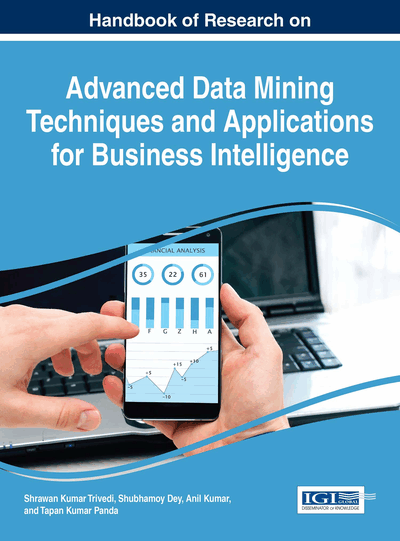# On Development of a Fuzzy Stochastic Programming Model with Its Application to Business Management

Animesh Biswas (University of Kalyani, India) and Arnab Kumar De (Government College of Engineering and Textile Technology Serampore, India)
DOI: 10.4018/978-1-5225-2031-3.ch021

## Abstract

This chapter expresses efficiency of fuzzy goal programming for multiobjective aggregate production planning in fuzzy stochastic environment. The parameters of the objectives are taken as normally distributed fuzzy random variables and the chance constraints involve joint Cauchy distributed fuzzy random variables. In model formulation process the fuzzy chance constrained programming model is converted into its equivalent fuzzy programming using probabilistic technique, a-cut of fuzzy numbers and taking expectation of parameters of the objectives. Defuzzification technique of fuzzy numbers is used to find multiobjective linear programming model. Membership function of each objective is constructed depending on their optimal values. Afterwards a weighted fuzzy goal programming model is developed to achieve the highest degree of each of the membership goals to the extent possible by minimizing group regrets in a multiobjective decision making context. To explore the potentiality of the proposed approach, production planning of a health drinks manufacturing company has been considered.
Chapter Preview
Top

## Introduction

In today’s highly competitive, fluctuating complex organizational situation, organizations around the world have increasingly emphasized on aggregate production planning (APP) for determining a best way to achieve the highest possible extent of a set of objectives in an environment of conflicting interests, incomplete information and limited resources as studied by Simon (1977). APP deals with matching supply and demand of forecasted and fluctuated customer’s orders over the period of medium time range. The problem of APP is concerned with management’s response to fluctuations in the demand pattern. Specifically, how can the productive, man power, goods resources and other controllable factors can be utilized effectively in the face of changing demands in order to minimize the total cost of operations over a given planning horizon (Nam & Logendran, 1992; Buxey, 1995).

The APP problems have been extensively investigated and much effort has been expended in developing decision models (Saad, 1982). Thereafter, many researchers studied APP problems and developed different optimization models (Jain & Palekar, 2005; Gomes da Silva et al., 2006; Leung et al., 2006; Leung & Chan, 2009). Most of the above models were developed under crisp environments, and can be categorized as deterministic optimization models. However, it is frequently observed that the APP problems contain many uncertain factors, viz., customer demand, production fluctuation, and their associated costs (Mula et al. 2006). In particular, in many practical applications, the imprecise information embedded in APP can be obtained subjectively (Wang & Liang, 2004; Jamalnia & Soukhakian, 2009). Zadeh (1965), and Bellman and Zadeh (1970) introduced fuzzy set theory to handle uncertainties of this type. After the pioneering work on fuzzy linear programming (FLP) by Tanaka et al. (1973) and Zimmermann (1978), several kinds of FLP models appeared in the literature and different methods have been proposed to solve such problems. Some methods are based on the concepts of the penalty method (Jamison & Lodwick, 2001), the satisfaction degree of the constraints (Liu, 2001), the statistical confidence interval (Chiang, 2001), and the multiobjective optimization method (Zhang et al, 2003). Other kinds of methods are semi-infinite programming method (Leon & Vercher, 2004), the superiority and inferiority of fuzzy numbers (FNs) (Hop, 2007), and the degrees of feasibility (Jimenez et al, 2007). Ganesan and Veeramani (2006) discussed a method for solving FLP problems without converting them to crisp linear programming problems. There have been relatively few studies of fuzzy APP problems (Lai & Hwang 1992). Since the APP problem in crisp environments can be modelled as a linear program, on the basis of FLP (Zimmermann 1976) several fuzzy optimization methods for solving fuzzy APP problems have been developed (Rommelfanger 1996). Rinks (1982) investigated APP problems based on fuzzy logic and fuzzy linguistics and developed the fuzzy APP model and algorithm, and examined the robustness of this model under varying cost structures. Lee (1990) discussed the APP problems for a single product type with a fuzzy objective, fuzzy workforce levels, and fuzzy demands. Gen et al. (1992) proposed a method for solving the APP problem with multiple objectives and fuzzy parameters, and provided interactive solutions that suggest the best compromise aggregate production plan. Tang et al. (2000) developed an approach to multi-product APP problems with fuzzy demands and capacities. Afterwards, Tang et al. (2003) focused on the simulation analysis of the APP problems of this kind. There are several other related studies, including the approaches developed by Wang and Fang (2001), and Wang and Liang (2005).

## Complete Chapter List

Search this Book:
Reset